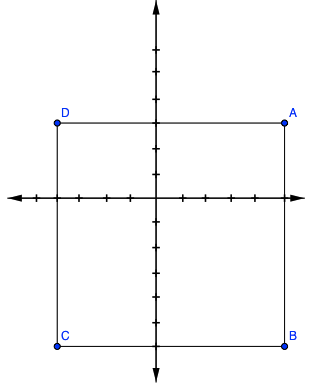### Home > GC > Chapter 1 > Lesson 1.2.3 > Problem1-73

1-73.

Plot the following points on another sheet of graph paper and connect them in the order given. Then connect points $A$ and $D$.

$A(5,3)$, $B(5,-6)$, $C(-4,-6)$, and $D(-4,3)$

1. What is the resulting figure?

2. Find the area of shape $ABCD$.

3. If $ABCD$ is rotated $90°$clockwise ($\circlearrowright$) about the origin to form $A'B'C'D'$, what are the coordinates of the vertices of $A'B'C'D'$?$A'(3,−5)$, $B'(-6,-5)$, $C'\left(-6,4\right)$$D'(3,4)$